Home
Hostname: page-component-7ccbd9845f-9nx8b Total loading time: 1.048 Render date: 2023-01-27T18:59:53.666Z Has data issue: true Feature Flags: { "useRatesEcommerce": false } hasContentIssue true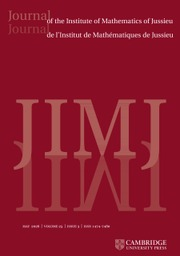Journal of the Institute of Mathematics of Jussieu

# TEST IDEALS IN RINGS WITH FINITELY GENERATED ANTI-CANONICAL ALGEBRAS

Published online by Cambridge University Press:  13 January 2016

## Abstract

Many results are known about test ideals and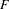$F$-singularities for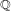$\mathbb{Q}$-Gorenstein rings. In this paper, we generalize many of these results to the case when the symbolic Rees algebra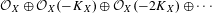${\mathcal{O}}_{X}\oplus {\mathcal{O}}_{X}(-K_{X})\oplus {\mathcal{O}}_{X}(-2K_{X})\oplus \cdots \,$ is finitely generated (or more generally, in the log setting for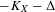$-K_{X}-\unicode[STIX]{x1D6E5}$). In particular, we show that the$F$-jumping numbers of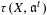$\unicode[STIX]{x1D70F}(X,\mathfrak{a}^{t})$ are discrete and rational. We show that test ideals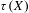$\unicode[STIX]{x1D70F}(X)$ can be described by alterations as in Blickle–Schwede–Tucker (and hence show that splinters are strongly$F$-regular in this setting – recovering a result of Singh). We demonstrate that multiplier ideals reduce to test ideals under reduction modulo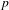$p$ when the symbolic Rees algebra is finitely generated. We prove that Hartshorne–Speiser–Lyubeznik–Gabber-type stabilization still holds. We also show that test ideals satisfy global generation properties in this setting.

Type
Research Article
Information

## Access options

Get access to the full version of this content by using one of the access options below. (Log in options will check for institutional or personal access. Content may require purchase if you do not have access.)

## References

Aberbach, I. M. and MacCrimmon, B., Some results on test elements, Proc. Edinb. Math. Soc. (2) 42(3) (1999), 541549, MR 1721770 (2000i:13005).CrossRefGoogle Scholar
Bhatt, B., Derived splinters in positive characteristic, Compos. Math. 148(6) (2012), 17571786, MR 2999303.CrossRefGoogle Scholar
Birkar, C., Existence of flips and minimal models for 3-folds in char$p$ , Annales Scientifiques de l’ENS, to appear, 2013, arXiv:1311.3098.Google Scholar
Birkar, C., Cascini, P., Hacon, C. D. and McKernan, J., Existence of minimal models for varieties of log general type, J. Amer. Math. Soc. 23(2) (2010), 405468, MR 2601039 (2011f:14023).CrossRefGoogle Scholar
Birkar, C. and Waldron, J., Existence of Mori fibre spaces for 3-folds in char$p$ , Preprint, 2014, arXiv:1410.4511.Google Scholar
Blickle, M., Test ideals via algebras of p -e -linear maps, J. Algebraic Geom. 22(1) (2013), 4983, MR 2993047.CrossRefGoogle Scholar
Blickle, M., Mustaţǎ, M. and Smith, K. E., Discreteness and rationality of F-thresholds, Michigan Math. J. 57 (2008), 4361, special volume in honor of Melvin Hochster MR 2492440 (2010c:13003).CrossRefGoogle Scholar
Blickle, M., Mustaţă, M. and Smith, K. E., F-thresholds of hypersurfaces, Trans. Amer. Math. Soc. 361(12) (2009), 65496565, MR 2538604 (2011a:13006).CrossRefGoogle Scholar
Blickle, M. and Schwede, K., p -1 -linear maps in algebra and geometry, in Commutative Algebra, pp. 123205 (Springer, New York, 2013).CrossRefGoogle Scholar
Blickle, M., Schwede, K., Takagi, S. and Zhang, W., Discreteness and rationality of F-jumping numbers on singular varieties, Math. Ann. 347(4) (2010), 917949, MR 2658149.CrossRefGoogle Scholar
Blickle, M., Schwede, K. and Tucker, K., F-singularities via alterations, Amer. J. Math. 137(1) (2015), 61109, MR 3318087.CrossRefGoogle Scholar
Boucksom, S., de Fernex, T., Favre, C. and Urbinati, S., Valuation spaces and multiplier ideals on singular varieties, in Recent Advances in Algebraic Geometry, London Math. Soc. Lecture Note Ser., Volume 417, pp. 2951 (Cambridge University Press, Cambridge, 2015), MR 3380442.CrossRefGoogle Scholar
Cascini, P., Tanaka, H. and Xu, C., On base point freeness in positive characteristic, Preprint, 2013, arXiv:1305.3502.Google Scholar
Chiecchio, A., About a Minimal Model Program without flips, Preprint, 2014, arXiv:1410.6230.Google Scholar
Chiecchio, A. and Urbinati, S., Ample Weil divisors, J. Algebra 437 (2015), 202221, MR 3351963.CrossRefGoogle Scholar
Cutkosky, S., Weil divisors and symbolic algebras, Duke Math. J. 57(1) (1988), 175183, MR 952230 (89e:14003).CrossRefGoogle Scholar
de Fernex, T., Docampo, R., Takagi, S. and Tucker, K., Comparing multiplier ideals to test ideals on numerically ℚ-Gorenstein varieties, Bull. Lond. Math. Soc. 47(2) (2015), 359369, MR 3335128.CrossRefGoogle Scholar
De Fernex, T. and Hacon, C., Singularities on normal varieties, Compos. Math. 145(2) (2009), 393414.CrossRefGoogle Scholar
Demazure, M., Anneaux gradués normaux, in Introduction à la théorie des singularités, II, Travaux en Cours, Volume 37, pp. 3568 (Hermann, Paris, 1988).Google Scholar
Ein, L., Lazarsfeld, R., Smith, K. E. and Varolin, D., Jumping coefficients of multiplier ideals, Duke Math. J. 123(3) (2004), 469506, MR 2068967 (2005k:14004).CrossRefGoogle Scholar
Fujino, O., Schwede, K. and Takagi, S., Supplements to non-lc ideal sheaves, in Higher Dimensional Algebraic Geometry, pp. 147 (Res. Inst. Math. Sci. (RIMS), Kyoto, 2011).Google Scholar
Gabber, O., Notes on some t-structures, in Geometric aspects of Dwork theory. Vol. I, II, pp. 711734 (Walter de Gruyter GmbH & Co. KG, Berlin, 2004).Google Scholar
Goto, S., Herrmann, M., Nishida, K. and Villamayor, O., On the structure of Noetherian symbolic Rees algebras, Manuscripta Math. 67(2) (1990), 197225, MR 1042238 (91a:13006).CrossRefGoogle Scholar
Hacon, C. D. and Xu, C., On the three dimensional minimal model program in positive characteristic, J. Amer. Math. Soc. 28(3) (2015), 711744, MR 3327534.CrossRefGoogle Scholar
Hara, N., Geometric interpretation of tight closure and test ideals, Trans. Amer. Math. Soc. 353(5) (2001), 18851906, (electronic) MR 1813597 (2001m:13009).CrossRefGoogle Scholar
Hara, N. and Yoshida, K.-I., A generalization of tight closure and multiplier ideals, Trans. Amer. Math. Soc. 355(8) (2003), 31433174, (electronic) MR 1974679 (2004i:13003).CrossRefGoogle Scholar
Hartshorne, R., Algebraic Geometry, Graduate Texts in Mathematics, No. 52, (Springer-Verlag, New York, 1977), MR 0463157 (57 #3116).CrossRefGoogle Scholar
Hartshorne, R., Generalized divisors on Gorenstein schemes, in Proceedings of Conference on Algebraic Geometry and Ring Theory in honor of Michael Artin, Part III (Antwerp, 1992), Volume 8, pp. 287339. (1994).Google Scholar
Hartshorne, R. and Speiser, R., Local cohomological dimension in characteristic p , Ann. of Math. (2) 105(1) (1977), 4579, MR 0441962 (56 #353).CrossRefGoogle Scholar
Hochster, M., Foundations of tight closure theory, in Lecture Notes from a Course Taught on the University of Michigan Fall 2007 (2007).Google Scholar
Hochster, M. and Huneke, C., Tight closure, invariant theory, and the Briançon–Skoda theorem, J. Amer. Math. Soc. 3(1) (1990), 31116, MR 1017784 (91g:13010).Google Scholar
Hochster, M. and Huneke, C., Infinite integral extensions and big Cohen–Macaulay algebras, Ann. of Math. (2) 135(1) (1992), 5389, MR 1147957 (92m:13023).CrossRefGoogle Scholar
Hochster, M. and Huneke, C., F-regularity, test elements, and smooth base change, Trans. Amer. Math. Soc. 346(1) (1994), 162, MR 1273534 (95d:13007).Google Scholar
Huneke, C. and Lyubeznik, G., Absolute integral closure in positive characteristic, Adv. Math. 210(2) (2007), 498504, MR 2303230 (2008d:13005).CrossRefGoogle Scholar
de Jong, A. J., Smoothness, semi-stability and alterations, Inst. Hautes Études Sci. Publ. Math. (83) (1996), 5193, MR 1423020 (98e:14011).Google Scholar
Katzman, M., Lyubeznik, G. and Zhang, W., On the discreteness and rationality of F-jumping coefficients, J. Algebra 322(9) (2009), 32383247, MR 2567418 (2011c:13005).CrossRefGoogle Scholar
Katzman, M., Schwede, K., Singh, A. K. and Zhang, W., Rings of Frobenius operators, Math. Proc. Cambridge Philos. Soc. 157(1) (2014), 151167, MR 3211813.CrossRefGoogle Scholar
Kollár, J., Exercises in the birational geometry of algebraic varieties, Preprint, 2008,arXiv:0809.2579.Google Scholar
Kollár, J. and Mori, S., Birational geometry of algebraic varieties, Cambridge Tracts in Mathematics, Volume 134 (Cambridge University Press, Cambridge, 1998), With the collaboration of C. H. Clemens and A. Corti, Translated from the 1998 Japanese original MR 1658959 (2000b:14018).CrossRefGoogle Scholar
Kunz, E., On Noetherian rings of characteristic p , Amer. J. Math. 98(4) (1976), 9991013, MR 0432625 (55 #5612).CrossRefGoogle Scholar
Lazarsfeld, R., Positivity in Algebraic Geometry. I, in Ergebnisse der Mathematik und ihrer Grenzgebiete. 3. Folge. A Series of Modern Surveys in Mathematics [Results in Mathematics and Related Areas. 3rd Series. A Series of Modern Surveys in Mathematics], Classical Setting: Line Bundles and Linear Series, Volume 48 (Springer-Verlag, Berlin, 2004), MR 2095471 (2005k:14001a).Google Scholar
Lipman, J., Rational singularities, with applications to algebraic surfaces and unique factorization, Inst. Hautes Études Sci. Publ. Math. (36) (1969), 195279, MR 0276239 (43 #1986).CrossRefGoogle Scholar
Lyubeznik, G., F-modules: applications to local cohomology and D-modules in characteristic p > 0, J. Reine Angew. Math. 491 (1997), 65130, MR 1476089 (99c:13005).Google Scholar
Lyubeznik, G. and Smith, K. E., Strong and weak F-regularity are equivalent for graded rings, Amer. J. Math. 121(6) (1999), 12791290, MR 1719806 (2000m:13006).CrossRefGoogle Scholar
Lyubeznik, G. and Smith, K. E., On the commutation of the test ideal with localization and completion, Trans. Amer. Math. Soc. 353(8) (2001), 31493180, (electronic) MR 1828602 (2002f:13010).CrossRefGoogle Scholar
Mustaţă, M., The non-nef locus in positive characteristic, in A Celebration of Algebraic Geometry, Clay Math. Proc., Volume 18, pp. 535551 (Amer. Math. Soc., Providence, RI, 2013).Google Scholar
Pérez, F., On the constancy regions for mixed test ideals, J. Algebra 396 (2013), 8297, MR 3108073.CrossRefGoogle Scholar
Schwede, K., F-adjunction, Algebra Number Theory 3(8) (2009), 907950, MR 2587408 (2011b:14006).CrossRefGoogle Scholar
Schwede, K., Test ideals in non-ℚ-Gorenstein rings, Trans. Amer. Math. Soc. 363(11) (2011), 59255941.CrossRefGoogle Scholar
Schwede, K. and Smith, K. E., Globally F-regular and log Fano varieties, Adv. Math. 224(3) (2010), 863894, MR 2628797 (2011e:14076).CrossRefGoogle Scholar
Schwede, K. and Tucker, K., On the behavior of test ideals under finite morphisms, J. Algebraic Geom. 23(3) (2014a), 399443, MR 3205587.CrossRefGoogle Scholar
Schwede, K. and Tucker, K., Test ideals of non-principal ideals: Computations, jumping numbers, alterations and division theorems, J. Math. Pures Appl. (9) 102(5) (2014b), 891929, MR 3271293.CrossRefGoogle Scholar
Schwede, K., Tucker, K. and Zhang, W., Test ideals via a single alteration and discreteness and rationality of F-jumping numbers, Math. Res. Lett. 19(01) (2012), 191197.CrossRefGoogle Scholar
Singh, A. K., Q -Gorenstein splinter rings of characteristic p are F-regular, Math. Proc. Cambridge Philos. Soc. 127(2) (1999), 201205, MR 1735920 (2000j:13006).CrossRefGoogle Scholar
Singh, A. K., Private communication, 2001–2014.Google Scholar
Smith, K. E., The multiplier ideal is a universal test ideal, Comm. Algebra 28(12) (2000), 59155929, Special issue in honor of Robin Hartshorne MR 1808611 (2002d:13008).CrossRefGoogle Scholar
Takagi, S., An interpretation of multiplier ideals via tight closure, J. Algebraic Geom. 13(2) (2004), 393415, MR 2047704 (2005c:13002).CrossRefGoogle Scholar
Watanabe, K., Some remarks concerning Demazure’s construction of normal graded rings, Nagoya Math. J. 83 (1981), 203211, MR 632654 (83g:13016).CrossRefGoogle Scholar
Xu, C., On the base-point-free theorem of 3-folds in positive characteristic, J. Inst. Math. Jussieu 14(3) (2015), 577588, MR 3352529.CrossRefGoogle Scholar

5
Cited by

# Save article to Kindle

Note you can select to save to either the @free.kindle.com or @kindle.com variations. ‘@free.kindle.com’ emails are free but can only be saved to your device when it is connected to wi-fi. ‘@kindle.com’ emails can be delivered even when you are not connected to wi-fi, but note that service fees apply.

Find out more about the Kindle Personal Document Service.

TEST IDEALS IN RINGS WITH FINITELY GENERATED ANTI-CANONICAL ALGEBRAS
Available formats
×

# Save article to Dropbox

To save this article to your Dropbox account, please select one or more formats and confirm that you agree to abide by our usage policies. If this is the first time you used this feature, you will be asked to authorise Cambridge Core to connect with your Dropbox account. Find out more about saving content to Dropbox.

TEST IDEALS IN RINGS WITH FINITELY GENERATED ANTI-CANONICAL ALGEBRAS
Available formats
×

# Save article to Google Drive

To save this article to your Google Drive account, please select one or more formats and confirm that you agree to abide by our usage policies. If this is the first time you used this feature, you will be asked to authorise Cambridge Core to connect with your Google Drive account. Find out more about saving content to Google Drive.

TEST IDEALS IN RINGS WITH FINITELY GENERATED ANTI-CANONICAL ALGEBRAS
Available formats
×
×